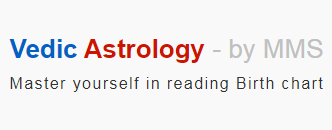Ascendant Ascendant : We know that Ascendant in a horoscope indicates the time of birth of a person. The Zodiacal sign that the Sun was rising in the Eastern horizon at the time of birth of a person is known as Ascendant sign of the person. On any given day, all the planets will be almost in the same sign. But, only Ascendant will keep changing the signs for every two hours, approx. As all the houses are calculated starting from Ascendant, natural benefic may become functional malefic or Badhakathipathi and natural malefic may become functional benefic depending on the the placement of Ascendant. Similarly, Yoga may become Dosha and Dosha may become Yoga based on the Ascendant Sign. Such is the importance of Ascendant sign. Now, let us discuss in detail how Ascendant is determined based on Month and Time of birth, and Sunrise time at the place of birth. We know Sun will be traversing through one Rasi or Sign in each Tamil month. The First day of the Year or New Year is celebrated when Sun enters Aries in Chittirai Month. So, obviously Chittirai will be the first of 12 months. Going by this, the Sun, which was in Aries on the first day of Chittirai, will be in Taurus in Vaikasi, in Gemini in Aani and finally in Pisces in Panguni by moving one degree each day. The picture below shows in which Sign the Sun will be in each month. As the English months span across two months, it is better to determine the Ascendant sign using Tamil months.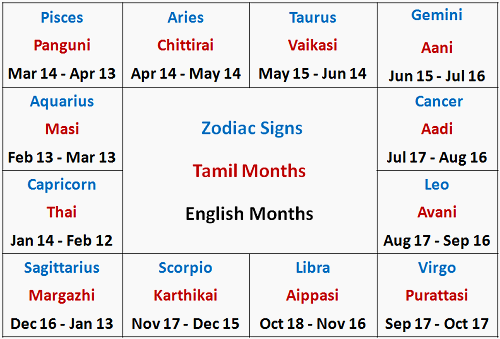We know the Earth takes 24 hours or 1440 minutes to rotate 360 degrees on its axis. So, for one degree it will be 1440 / 360 = 4 minutes. As each sign represents 30 degrees, the time taken by the Earth to rotate one Sign = 30 x 4 = 120 minutes i.e., 2 Hours. When the Sun rises at where we are on earth, then we will be in the same Sign as Sun will be. As the Earth rotates, the Sun will be rising in subsequent Signs after every 2 hours as the times go by. Now, let us take Avani (Aug 17th - Sep 16th). The Sun will be in Leo during this month. When the Sun rises at where we are, we too will be in Leo sign. As the Earth rotates, the Sun will be rising (ascending) in Virgo after 2 hours, in Libra after 4 hours and so on. At end of 24th hour, i.e., just before the Sunrise, it will be in Cancer. Again, on the next day morning, it will be in rising in Leo. In Vedic astrology, a "Day" means the time elapsed between two subsequent Sunrise time. For instance, if the Sunrise time is 5.57 am today, then starting from 5.57 am today to 5.57 am tomorrow is considered as One day. The Sunrise time can be obtained from Phanchang (Ephemeris) or e-Panchange or daily calender. Now, let us find out how to calculate the Ascendant sign using all these information. Let us say a person is born at 7.00 am in Avani month. And let the Sunrise time be 5.57 am on that day. As the month is Avani, Sun will be in Leo for the first 2 hours i.e., from 5.57 am to 7.56 am. As the person is born at 7.00 am, the Ascendant should be marked in Leo sign. But, if the person is born at 10.00 am in Avani, then Ascendant will be in Libra. It is because the Sun will be in Leo between 5.57 am to 7.56 am, in Virgo between 7.57 am to 9.56 am and in Libra between 9.57 am to 11.56 am. So, the Ascendant should be marked in Libra as the Sun will be in Libra at 10.00 am.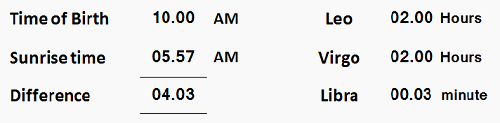This is a rough method of determining the Ascendant Sign because the Date of Birth is not accounted for. Moreover, Earth takes less than 2 hours to rotate some signs, and takes longer than 2 hours to rotate some signs. Now, let us find out how to determine the Ascendant sign precisely considering all these factors. As the Sun traverse every day, it will be at 1st degree of the Sign on Day 1 of the month, at 15th degree on Day 15th and at 30th degree on Day 30th. So, on the last day of the month, the time required by the Earth to rotate the Sign will be 1 degree x 4 min = 4 minutes only. For instance, on 1st of Chittirai, Earth will take 31 x 4 = 124 minutes to rotate the Aries sign. If it is 16th morning, then 16 x 4 = 64 min. And, if it is 31st morning, then Earth will take 1 x 4 = 4 min only to rotate the Aries sign.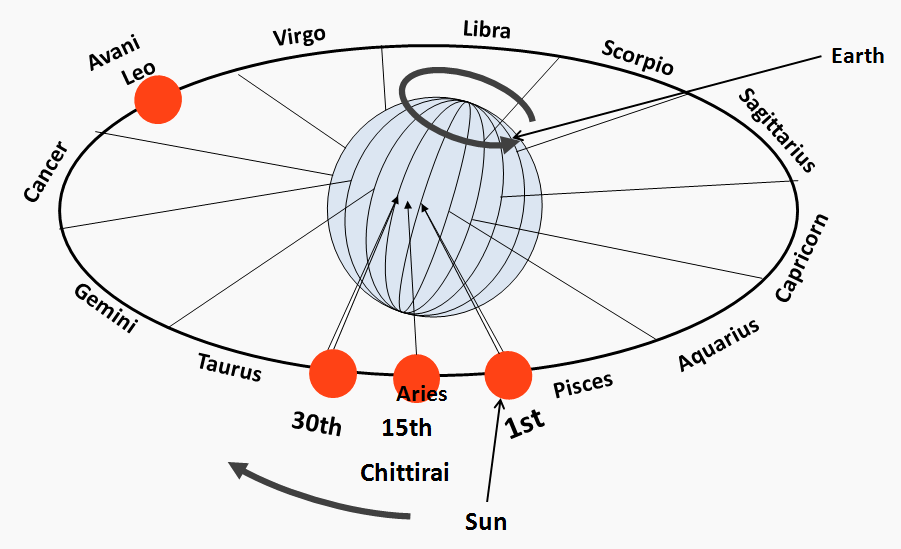As the Earth is tilted at 23.5 degree on its axis, the time taken by the Earth to rotate each sign will differ. The time taken by the Earth to rotate each Sign is called "Rasimanam" in Tamil, meaning "Measurement of Sign". The Rasimanam or the Rotation-Time is given below, both in Nazhigai and Minutes. The Sum total of minutes will be 1440 which is nothing but 24 hours. Similarly, the Sum total of Nazhigai will be 60 which makes one day.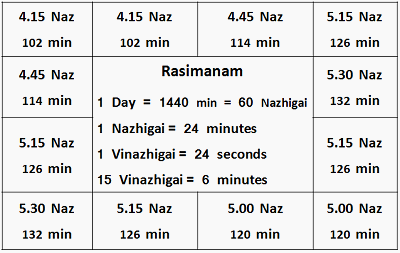Now, let us determine the Ascendant sign based on the Date, Time and Place of birth, Sunrise time and applying Rasimanam or the Rotation-Time of Sign. For this, First, find out the Elapsed Time (ET) between Sunrise Time (SrT) and Time of Birth (TOB) in minutes. Next, calculate the time required by the Earth to rotate the First Sign. Time required to rotate the other signs should be taken from the table Finally, subtract the Rotation-time of each Sign from ET until it cannot be subtracted. The Sign whose Rotation-Time cannot be subtracted will be the Ascendant Sign Ex 1 - Let us consider the DOB and TOB of a person is Aug 17th or Avani 1st, 10.00 AM. And let the Sunrise time be 5.57 AM on that day. ET = TOB - SrT = 10.00 - 05.57 = 4.03 Hrs = 4 x 60 + 3 = 243 min As the person is born on 1st Avani, the balance days in Avani is 31. Earth Rotation-time for the First sign Leo will be = 31/31 x 126 = 126 min 243 - 126 (First sign Leo) = 117 min. The Rotation-time for the next Sign Virgo is 120 min. As 120 cannot be subtracted from 117, the Ascendant Sign will be Virgo Ex 2 - Let us consider the Date and Time of birth of a person is Avani 16th, 10.00 AM. And let the Sunrise time be 6.01 AM on that day. Sun will be in Leo as the month is Avani. No of Days in Avani is 31. ET = TOB - SrT = 10.00 - 6.01 = 3.59 Hrs = 3 x 60 + 59 = 239 min As the Date of birth is 16th morning, the balance days in Avani is 31 - 15 = 16. Earth Rotation-time for the First sign Leo will be = 16/31 x 126 = 65.03 = 65 min Subtracting the Rotation-time for the First sign Leo and the next Sign Virgo, the balance time = 239 - (65.03+120) = 54 min. The Rotation time for the next Sign Libra time is 120 min. As 120 cannot be subtracted from 54, the Ascendant Sign will be Libra Ex 3 - Let us consider the Date and Time of birth of a person is Avani 31st, 6.05 PM. And let the Sunrise time be 6.05 AM on that day. ET = TOB - SrT = 18.05 - 6.05 = 12.00 Hrs = 12 x 60 = 720 min As the Date of birth is 31st evening, the balance days in Avani is just 0.5 days. Earth Traverse-time for the First sign Leo will be = 0.5/31 x 126 = 2.03 = 2 min Balance time = 720 - 2 (Leo) - 120 (Virgo) - 120 (Libra) - 126 (Scorpio) - 132 (Sagittarius) - 126 (Capricorn) = 94 min. The next Sign Aquarius time is 114 min. As 114 cannot be subtracted from 94, the Ascendant Sign will be Aquarius Now that we know how to determine the Ascendant sign, it is always better to ensure it is marked correctly in the horoscope because the entire forecast depends on the Ascendant. As a start, you can verify whether the Ascendant is correctly marked in your horoscope according to your Date, Time and Place of birth and Sunrise time at your place of birth.